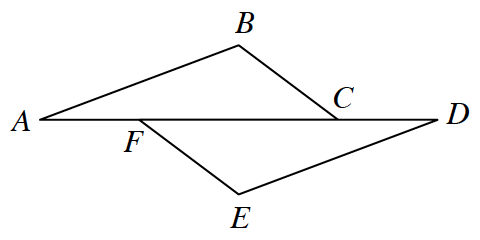### Home > INT2 > Chapter 7 > Lesson 7.2.1 > Problem7-73

7-73.Suzette started a proof to show that if $\overline{BC} // \overline{EF}$, $\overline{AB} // \overline{DE}$, and $AF = DC$, then $BC ≅ EF$. Examine her work below. Then complete her missing statements and reasons.

Statements

Reasons

1. $\overline{BC} // \overline{EF}$

1. $m∠BCF=m∠EFC$

1. $\overline{AB}//\overline{DE}$

1. $m∠BAC=m∠EDF$

1. $AF=DC$

Reflexive Property

1. $AF+FC=FC+DC$

Addition Property of Equality (adding the same amount to both sides of an equation keeps the equation true)

1. $AC=DF$

1. $ΔABC≅Δ​DEF$
$≅Δs→≅\text{parts}$